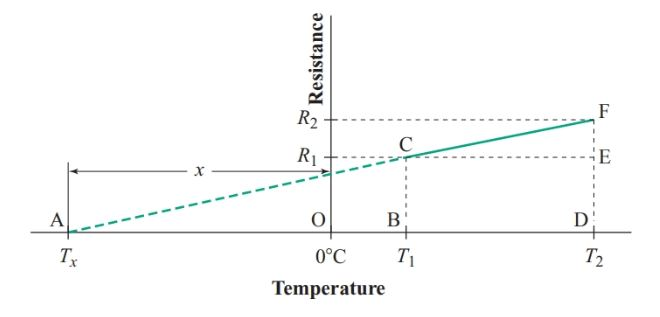Home / Basic Electrical / Effect of Temperature on Resistance | Temperature Coefficient of Resistance

# Effect of Temperature on Resistance | Temperature Coefficient of Resistance

Want create site? Find Free WordPress Themes and plugins.

Checking the voltage/current (V/I) ratio of conductors at various temperatures shows that the resistance of most conducting materials increases linearly with temperature except at very hot or very cold temperatures.

Temperature has little effect on the resistance of some alloys, such as constantan. For a few materials, including carbon and other semiconductors, the resistance decreases as the temperature increases.

Figure 1 shows the resistance of a conductor increases with temperature. R1 is the resistance at temperature T1, and R2 is the resistance at temperature T. The line segment CF shows how the resistance varies for temperatures between T1 and T2. The steeper the slope of segment CF, the more the resistance increases with temperature.Figure 1 Effect of temperature on resistance

Extending CF to the temperature axis produces triangles ABC and ADF. Since these triangles are similar,

$\frac{DF}{BC}=\frac{AD}{AB}=\frac{AO+OD}{AO+OB}$

Substituting the quantities that the sides of the triangles represent gives

$\begin{matrix} \frac{{{R}_{2}}}{{{R}_{1}}}=\frac{x+{{T}_{2}}}{x+{{T}_{1}}} & {} & \left( 1 \right) \\\end{matrix}$

Where R1 is the resistance of the conductor at temperature T1, R2 is the resistance at temperature T2, and x is the difference between 0°C and the temperature at which the resistance would be zero if it continued to decrease linearly at very low temperatures.

Table 1 lists values for x for some metals commonly used for conductors. The values for Nichrome II and constantan show that x is not the actual temperature at which the resistance becomes zero ohms. However, we can use x to calculate resistances within the range of temperatures where the resistance varies linearly with temperature.

 Material x(oC) Silver 243 Copper 234.5 Aluminum 236 Tungsten 202 Nickel 147 Iron 180 Nichrome 6 250 Constantan 125 000

Table 1 Values of x for common conductors

Example

A copper conductor has a resistance of 12.0 V at 20°C. Find the resistance at 100°C.

Solution

Since,

$\frac{{{R}_{2}}}{{{R}_{1}}}=\frac{x+{{T}_{2}}}{x+{{T}_{1}}}$

So,

${{R}_{2}}={{R}_{1}}\times \frac{x+{{T}_{2}}}{x+{{T}_{1}}}=12\Omega \times \frac{234.5+100}{234.5+20}=15.8\Omega$

Note that the temperature units, °C, cancel out in this calculation.

In addition to being able to calculate the effect that temperature has on resistance, we can use the change in resistance of a certain material to calculate the temperature in locations where it is difficult to place a thermometer.

Example

The copper winding of an electric motor that has been standing for several hours in a room at 20°C has a resistance of 0.20 V. When the motor has been in use for some hours, the resistance of the winding is found to be 0.22 V. Calculate the temperature rise in the winding.

Solution

\begin{align} & \frac{{{R}_{2}}}{{{R}_{1}}}=\frac{x+{{T}_{2}}}{x+{{T}_{1}}} \\ & \frac{0.22}{0.20}=\frac{234.5+{{T}_{2}}}{234.5+20} \\ & {{T}_{2}}=\frac{0.22}{0.20}\times \left( 234.5+20 \right)={{45.45}^{o}}C \\\end{align}

Temperature rise = T2 – T1 = 45.45oC – 20oC = 25oC

Substituting $R=\rho \frac{l}{A}$  for R1 in Equation 1 gives

$\begin{matrix} R=\rho \frac{l}{A}\left( \frac{x+T}{x+20} \right) & {} & \left( 2 \right) \\\end{matrix}$

With values for ρ and x, we can use Equation 2 to estimate the resistance of any metallic conductor at any temperature.

## Temperature Coefficient of Resistance

Now we shall derive an alternative method (Temperature Coefficient) of showing the effect of temperature on the resistance of a conductor. In Figure 1, line segment CE is parallel to the temperature axis. Since resistivity is usually stated for a temperature of 20°C, we shall let T1 represent 20°C.

Triangle ABC is similar to triangle CEF, so

$\frac{FE}{BC}=\frac{CE}{AB}$

Substituting the quantities that the sides of the triangles represent gives

$\frac{FE}{{{R}_{1}}}=\frac{\Delta T}{x+20}$

Where ΔT is the difference between T2 and 20°C.

Therefore,

$FE={{R}_{1}}\times \frac{\Delta T}{x+20}$

Since CE and BD are parallel,

${{R}_{2}}=ED+FE={{R}_{1}}+{{R}_{1}}\times \frac{\Delta T}{x+20}={{R}_{1}}\left( 1+\frac{\Delta T}{x+20} \right)$

The quantity $\frac{1}{x+20}$ is the temperature coefficient of resistance at 20°C, the proportion by which the resistance changes per degree of change in temperature from 20°C. We represent this coefficient by the Greek letter α (alpha).

$\begin{matrix} {{R}_{2}}={{R}_{1}}\left( 1+\alpha \Delta T \right) & {} & \left( 3 \right) \\\end{matrix}$

Where R2 is the resistance of a conductor at any specified temperature, R1 is its resistance at 20°C, α is the temperature coefficient of resistance at 20°C for the conductor, and ΔT is the difference between the specified temperature and 20°C.

Substituting ${{R}_{1}}=\rho \frac{l}{A}$ gives a general equation for the resistance of a conductor:

$R=\rho \frac{l}{A}\begin{matrix} \left( 1+\alpha \Delta T \right) & {} & \left( 4 \right) \\\end{matrix}$

where R is the resistance of the conductor in ohms, ρ is the resistivity of the material in ohmmeters at 20°C, l is the length in meters, A is the cross-sectional area in square meters, a is the temperature coefficient of resistance of the material at 20°C, and DT is the difference between the temperature of the conductor and 20°C.

Table 2 lists temperature coefficients for some materials commonly used for conductors and resistors.

 Conductor material α(K-1) Silver 0.003 8 Copper 0.003 93 Aluminum 0.003 9 Tungsten 0.004 5 Nickel 0.006 Iron 0.005 5 Nichrome 0.000 16 Constantan 0.000 008 Carbon -0.000 5

Table 2 Temperature coefficients of resistance at 20°C

Example

A heating element made from Nichrome II has a resistance of 16.0 V at 1500°C. Find the resistance at normal room temperature.

Solution

In this example, R2 in Equation 3 is given and R1 is unknown. Hence,

${{R}_{1}}=\frac{{{R}_{2}}}{1+\alpha \Delta T}=\frac{16}{1+0.00016\times 1480}=12.9\Omega$

If the required temperature is below 20°C, ΔT will be a negative quantity and the resulting resistance is less than the resistance at 20°C.

Did you find apk for android? You can find new Free Android Games and apps.